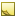# Aerodynamics and Propulsion

Coordinated flight is a condition in which the aircraft is being maintained with zero side-slip angle (and therefore zero aerodynamic side force). Under this condition, the aerodynamic and propulsion models provide a means to determine the orientation of the aircraft body axes (accounting for bank angle and angle of attack), aerodynamic forces, and propulsion forces required to execute the track and profile motion established by the maneuver.

See the Code Sample topic for sample code demonstrating how to use the coordinated flight condition with the aerodynamic an propulsion models to assess the body axes orientation of the aircraft, bank angle, angle of attack, aerodynamic and propulsion forces, throttle setting, and fuel flow rate.Note

The functionality described in this topic requires a license for the Aircraft Propagation Library.Aerodynamic Modeling

A SimpleFixedWingForwardFlightAerodynamics can be configured with the necessary parameters to define the lift and drag forces acting on the aircraft (the side force is zero by definition of this model).

The lift coefficient is determined from the coefficient value at zero angle of attack and the lift coefficient slope as a function of the angle of attack, α (in radians), according to the following relationship:

CL = CL0 + CLα α

The side coefficient is zero by definition of this model.

CS = 0

The drag coefficient is determined from the total minimum drag coefficient at zero lift, lift coefficient, aspect ratio (A), and the Oswald efficiency factor (e0) according to the following relationships:

CD = CDmin-total + CL2 / k;

k = π A e0

The total minimum drag coefficient is the sum of the nominal drag at zero lift plus an additional drag-count term.

CDmin-total = CD0 + 10-4 ΔCD

The aerodynamic forces are then the aerodynamic coefficients multiplied by the dynamic pressure and reference area.Propulsion Modeling

For propulsion, two models are available: SimpleForwardFlightPropellerPropulsion and SimpleForwardFlightJetPropulsion. These models, once configured with the necessary parameters, supply the power required (subject to the provided power/thrust available limits) and corresponding throttle setting and fuel flow rate.